Next: Rigid Body Rotation Up: Rotating Reference Frames Previous: Foucault Pendulum

# Exercises

1. A pebble is dropped down an elevator shaft in the Empire State Building (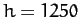ft, latitude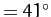N). Find the pebble's horizontal deflection (magnitude and direction) due to the Coriolis force at the bottom of the shaft. Neglect air resistance.

2. If a bullet is fired due east, at an elevation angle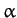, from a point on the Earth whose latitude is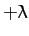show that it will strike the Earth with a lateral deflection. Is the deflection northward or southward? Here,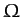is the Earth's angular velocity,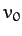is the bullet's initial speed, and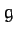is the acceleration due to gravity. Neglect air resistance.

3. A particle is thrown vertically with initial speed, reaches a maximum height, and falls back to the ground. Show that the horizontal Coriolis deflection of the particle when it returns to the ground is opposite in direction, and four times greater in magnitude, than the Coriolis deflection when it is dropped at rest from the same maximum height. Neglect air resistance.

4. The surface of the Diskworld is a disk which rotates (counter-clockwise looking down) with angular frequencyabout a perpendicular axis passing through its center. Diskworld gravitational acceleration is of magnitude, and is everywhere directed normal to the disk. A projectile is launched from the surface of the disk at a point whose radial distance from the axis of rotation is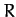. The initial velocity of the projectile (in a co-rotating frame) is of magnitude, is directly radially outwards, and is inclined at an angleto the horizontal. What are the radial and tangential displacements of the impact point from that calculated by neglecting the centrifugal and Coriolis forces? Neglect air resistance. You may assume that the displacements are small compared to bothand the horizontal range of the projectile.

5. Demonstrate that the Coriolis force causes conical pendulums to rotate clockwise and counter-clockwise with slightly different angular frequencies. What is the frequency difference as a function of terrestrial latitude?

6. A satellite is in a circular orbit of radiusabout the Earth. Let us define a set of co-moving Cartesian coordinates, centered on the satellite, such that the-axis always points toward the center of the Earth, the-axis in the direction of the satellite's orbital motion, and the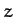-axis in the direction of the satellite's orbital angular velocity,. Demonstrate that the equation of motion of a small mass in orbit about the satellite are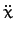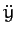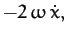assuming that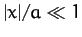and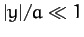. You may neglect the gravitational attraction between the satellite and the mass. Show that the mass executes a retrograde (i.e., in the opposite sense to the satellite's orbital rotation) elliptical orbit about the satellite whose period matches that of the satellite's orbit, and whose major and minor axes are in the ratio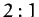, and are aligned along the- and-axes, respectively.Next: Rigid Body Rotation Up: Rotating Reference Frames Previous: Foucault Pendulum
Richard Fitzpatrick 2011-03-31